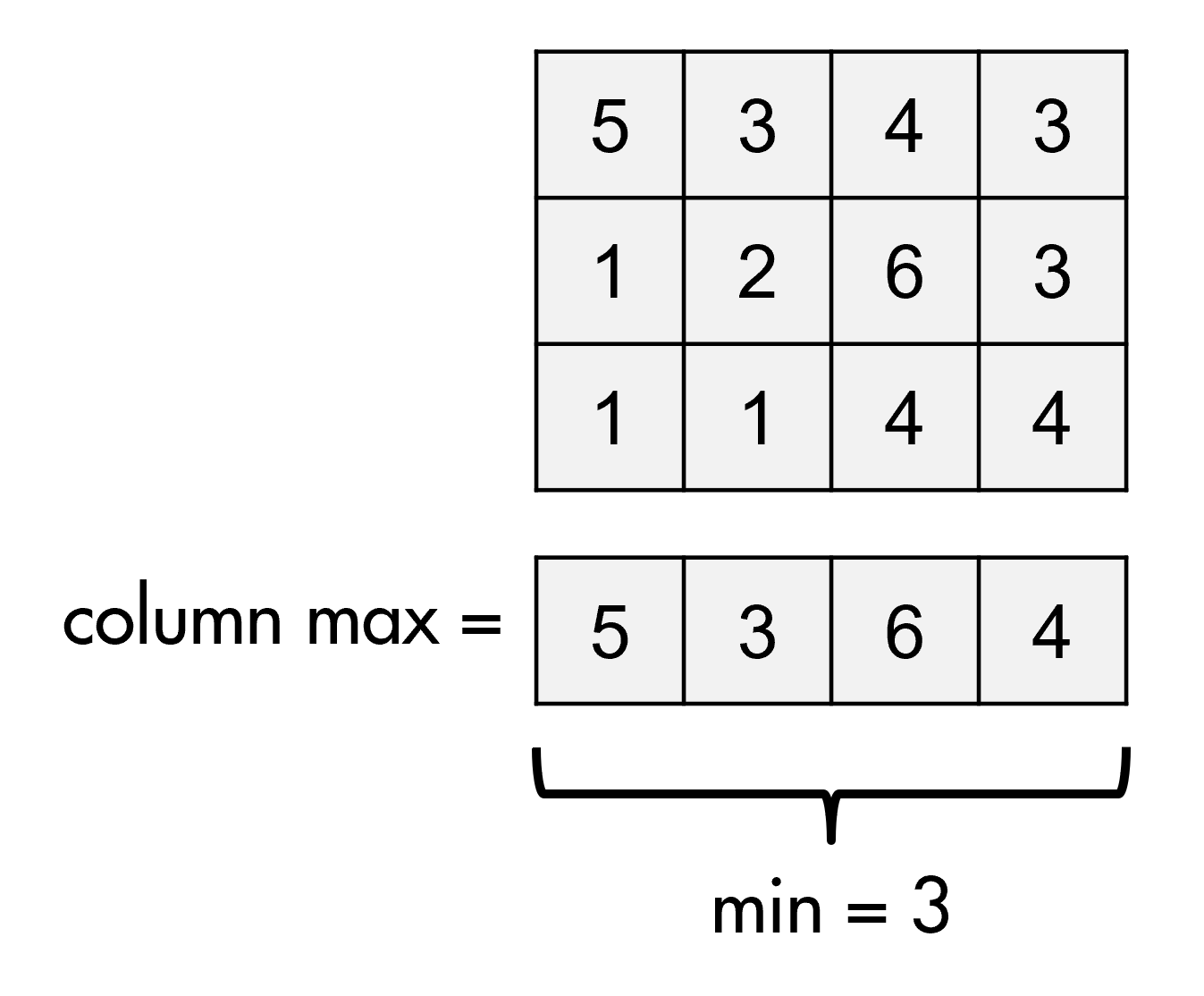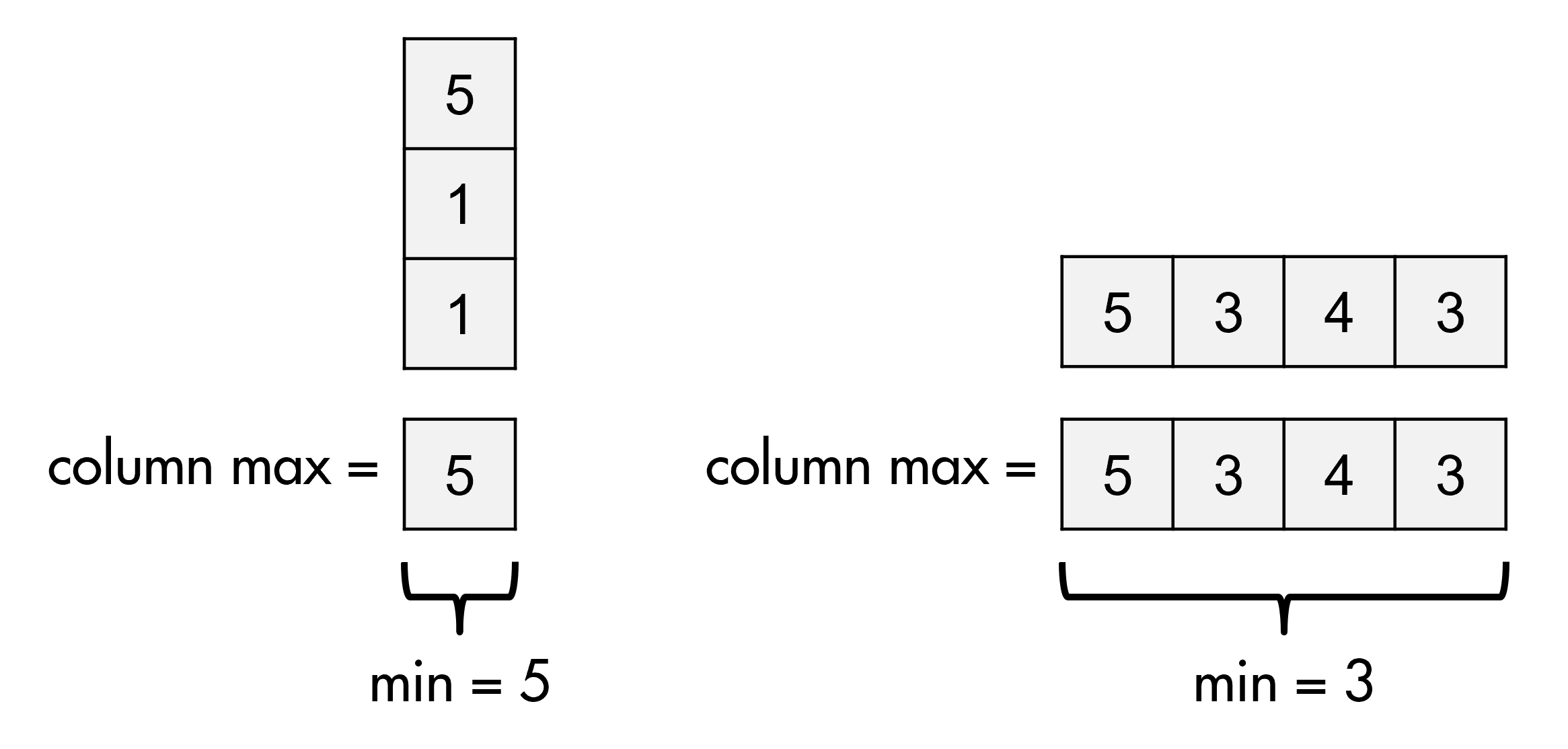# Problem 55250. Find the minimum of the column-maximums of a matrix

Given a matrix A, find the maximum value of each column, then return the smallest of those maximum values (ie return the minimum of the column maximums).A = [5 3 4 3;1 2 6 3;1 1 4 4];
m = minimax(A)
m =
3
Note that your function should work for matrices with one row or column (ie vectors) and scalars (in which case m = A).### Solution Stats

36.56% Correct | 63.44% Incorrect
Last Solution submitted on Dec 06, 2023

### Community Treasure Hunt

Find the treasures in MATLAB Central and discover how the community can help you!

Start Hunting!